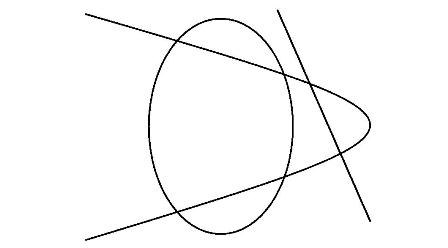Draw a ìcleanî scribble ñ one in which all regions are easily discernibleNow count:

 D Dots (where lines segments cross or the end of line segments) L Lines (line segment between 2 dots) R Regions (area surrounded by closed line segments)

For the scribble shown D = 10, L = 14 and R = 5

Investigate the formula connecting D, L and R.

### Hints

• Find a formula for a straight line with varying numbers of dots placed on it.
• Find a formula for regular polygons.
• Find a formula for a bicycle wheel with varying numbers of spokes.
• Consider other groups of scribbles.
• Formulate a formula for all scribbles.
• If you have a scribble which satisfies your formula, find the four simplest ways that the scribble can be made slightly larger.
• By carefully considering each of these changes, show that each of the larger scribbles also satisfies your formula.